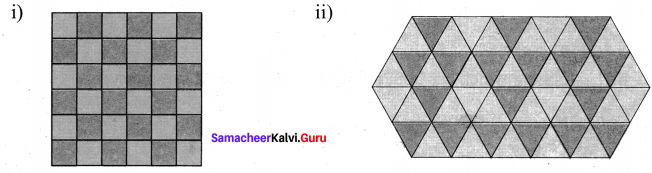# Samacheer Kalvi 6th Maths Solutions Term 3 Chapter 4 Geometry Ex 4.2## Tamilnadu Samacheer Kalvi 6th Maths Solutions Term 3 Chapter 4 Geometry Ex 4.2

Miscellaneous Practice problems

Question 1.
i) A triangle which has no line of symmetry
ii) A triangle which has only one line of symmetry
iii) A triangle which has three lines of symmetry
Solution:
(i) A Scalene triangle has no line of symmetry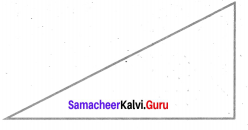(ii) An isosceles triangle has only one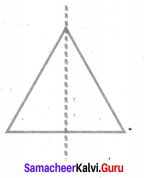(iii) An equilateral triangle has three lines of symmetry.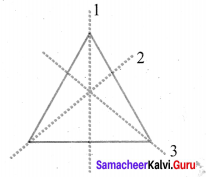Question 2.
Find the alphabets in the box which have
i) No Line of symmetry
ii) Rotational symmetry
iii) Reflection symmetry
iv) Reflection and rotational symmetry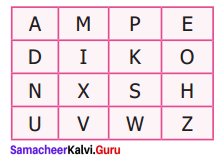Solution:
i) The alphabets which have no line of symmetry are P, N, S, Z
ii) The alphabets which have Rotational symmetry are I, O, N, X, S, H, Z
iii) The alphabets which have reflection symmetry are A, M, E, D, I, K, O, X, H, U, V, W.
iv) The alphabets which has reflection and rotational symmetry are I, O, X, H.Question 3.
For the following pictures, find the number of lines of symmetry and also find the order of rotation.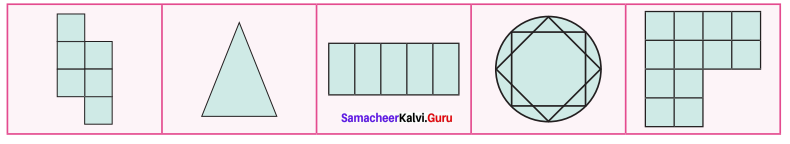Solution:
Number of lines of symmetry is 0; order of rotation is 2

ii)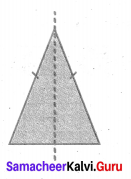Number of lines of symmetry is 1.
Order of rotation is 0, because it is an isosceles triangle

iii) Number of lines of symmetry are 2
Order of rotation is 2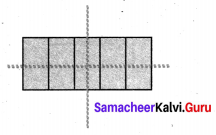iv) Number of lines of symmetry 8 and order of rotation 8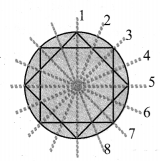v)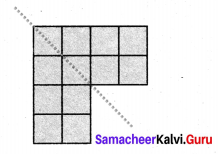Number of line of symmetry is 1 and order of rotation 0

Question 4.
The three digit number 101 has rotational and reflection symmetry. Give five more examples of three digit number which have both rotational and reflection symmetry.
Solution:
181, 111, 808, 818, 888Question 5.
Translate the given pattern and com ilete the design in rectangular strip?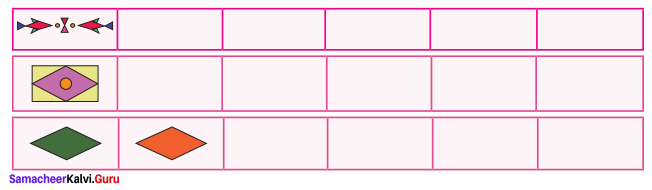Solution: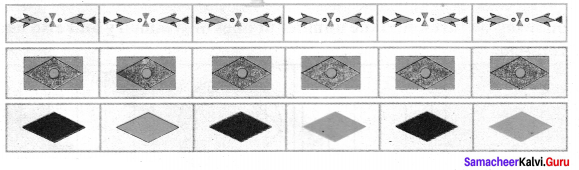Challenge Problems

Question 6.
Shade one square so that it possesses
i) One line of symmetry
ii) Rotational symmetry of order 2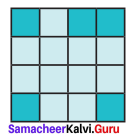Solution: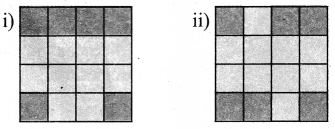Question 7.
Join six identical squares so that atleast one side of a square fits exactly with any other side of the square and have reflection symmetry (any three ways).
Solution:
The required combination of squares are given below.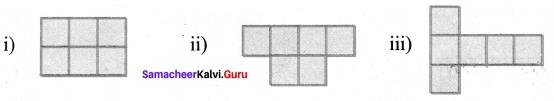Question 8.
Draw the following:
Solution:
i) A figure which has reflection symmetry but no rotational symmetry.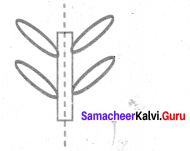ii) A figure which has rotational symmetry but no reflection symmetry.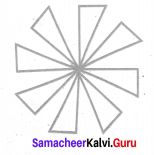iii) A figure which has both reflection and rotational symmetry.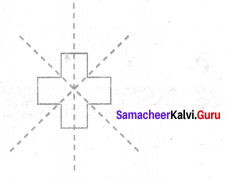Question 9.
Find the line of symmetry and the order of rotational symmetry of the given regular polygons and complete the following table and answer the questions given below.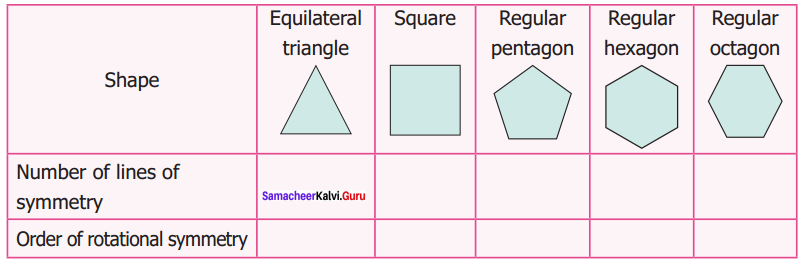i) A regular polygon of 10 sides will have ____ lines of symmetry.
ii) If a regular polygon has 10 lines of symmetry, then its order of rotational symmetry is _____
iii) A regular polygon of ‘n’ sides ______ has lines of symmetry and the order of rational symmery is _____.Question 10.
Color the boxes in such a way that it possess translation symmetry.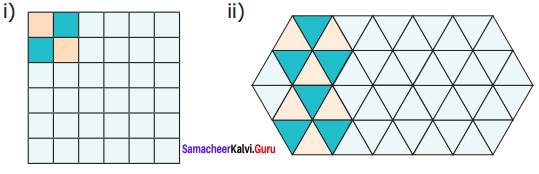Solution:
The following figures coloured to posses translation symmetry.